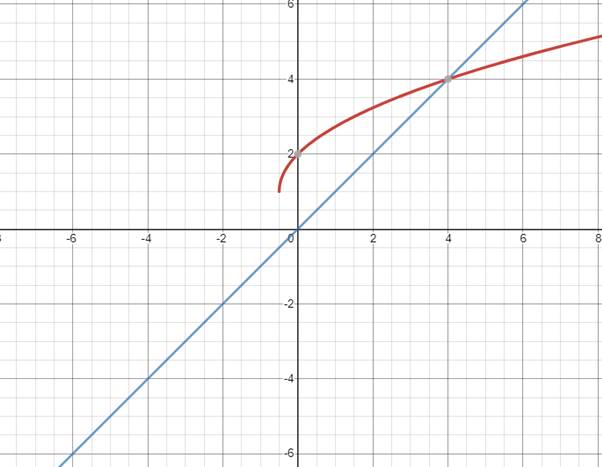# Find the solution of the equation using graph.### Precalculus: Mathematics for Calcu...

6th Edition
Stewart + 5 others
Publisher: Cengage Learning
ISBN: 9780840068071### Precalculus: Mathematics for Calcu...

6th Edition
Stewart + 5 others
Publisher: Cengage Learning
ISBN: 9780840068071

#### Solutions

Chapter 1.9, Problem 51E
To determine

## Find the solution of the equation using graph.

Expert Solution

x=4

### Explanation of Solution

Given information:

2x+1+1=x

2x+1+1=xHence the graph intersect at x=4 , the solution of the given equation is x=4 .

### Have a homework question?

Subscribe to bartleby learn! Ask subject matter experts 30 homework questions each month. Plus, you’ll have access to millions of step-by-step textbook answers!